## General Question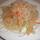# What is the formula for calculating the rate of water escaping through a hole in a pipe?

Asked by malevolentbutticklish (2155) March 17th, 2010

p = pressure in PSI of water in the pipe
d = diameter of the hole in inches

Assume the pipe is large enough that there is no pressure drop.

This isn’t a homework question. An actual pipe exists into which I am going to drill an actual hole.

Observing members: 0Composing members: 0## 12 Answers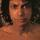I’m not entirely sure if this is what you are looking for, but it may be helpful.
http://www.1728.com/flowrate.htm

Scroll down past the calculator to the flow rate equation (# 3). Again, not sure if it’s exactly what you are looking for, but maybe you’ll get something out of it.

jeffgoldblumsprivatefacilities (19677)“Great Answer” (0) Flag as…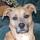Assuming the pipe currently has some kind of outlet (a tap, for instance) I’d do this by observation before attempting to calculate: Open the valve wide open for a minute and see how much water you collect in a minute. Compare that to the tap size, and make your calculations accordingly.

Most of the flow rate calculators I’ve seen don’t depend so much on “pressure” as “velocity”. That is, they’ll accept as input the velocity of the medium in your pipe (since flow rate calculations aren’t done on “water”, but on “whatever medium is in the pipe”, which could be air, oil, gas, sewage, etc.).

The other thing I wouldn’t be doing, I think, is drilling into a pipe full of water. I’d want to drain it first. Maybe that’s just me…

CyanoticWasp (20063)“Great Answer” (0) Flag as…42

erichw1504 (26398)“Great Answer” (2) Flag as…@jeffgoldblumsprivatefacilities: This is not what I am looking for. To use the calculator you must know two of [pipe diameter, velocity, flow rate]. I know pipe diameter (in this case the size of the hole drilled in the pipe NOT the pipe diameter) but I do not know how to calculate velocity or flow rate from pressure. Do you?

malevolentbutticklish (2155)“Great Answer” (1) Flag as…@CyanoticWasp: In my case you have to know the medium in the pipe. This is because you have to know the viscosity. A high-viscosity fluid under the same pressure will exit the same size hole more slowly. Consider how much faster water would come out a toothpaste tube than toothpaste if the tube was squeezed at equal pressure. (I know, Toothpaste is a colloid.) In this case trial and error would be a pain in the ass.

malevolentbutticklish (2155)“Great Answer” (1) Flag as…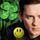Lee has a flow calculator .
I think that is what you need. Let us know.

LuckyGuy (36619)“Great Answer” (0) Flag as…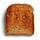I know my Pocket Ref has this formula in it, I’ll post it when I get home. (Don’t have it on me at the moment for once…)

ShiningToast (2101)“Great Answer” (0) Flag as…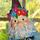Bernoulli applies for incompressible fluids, steady and inviscid flow, which sounds like your condition. Therefore:

P1 + .5*ρ*V1^2 + γ*z1 = P2 + .5*ρ*V2^2 + γ*z2

P = pressure
ρ = density of water
V = velocity
γ = specific weight of water
z = height

1 is an arbitrary starting point sufficiently far away from 2, the location of the hole, such that the velocity of water at point 1 is zero. The height is constant from 1 to 2, assuming horizontal pipe. You can say P2 is zero if you account for atmospheric pressure in P1. Therefore, the equation reduces to:

P1 = .5*ρ*V2^2

This does not account for friction losses at the hole.

You can relate the velocity of water to volumetric flow rate using Q=AV.

If the viscosity is significant, things get a little more complicated, but I have equations for laminar and turbulent conditions if you need it.

lilikoi (10079)“Great Answer” (2) Flag as…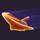When I did a problem like this I recall using Bernoulli as well.

Shuttle128 (2979)“Great Answer” (0) Flag as…Pocket Ref has just a table of values based upon pipe diameter/water jet length., so sorry I couldn’t help with the formula.

@lilikoi seems to know what is going on though.

ShiningToast (2101)“Great Answer” (0) Flag as…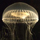Simple method. Count how many gallon jugs can be filled in 20 seconds. Multiply both by 3. You will get how many gallons per minute.

striveshare (1)“Great Answer” (0) Flag as…@striveshare I believe that he’s trying to calculate the amount before he drills a hole in the pipe and finds out via your empirical method.

CyanoticWasp (20063)“Great Answer” (1) Flag as…## Answer this questionor

#### Join

to answer.

This question is in the General Section. Responses must be helpful and on-topic.

Your answer will be saved while you login or join.
Have a question? Ask Fluther!
What do you know more about?
or
Knowledge Networking @ Fluther